# RD Sharma Solutions for Class 6 Maths Chapter 9: Ratio, Proportion and Unitary Method Objective Type Questions

In our day to day life, we often come across situations where we need to compare quantities in terms of their magnitudes/ measurements. RD Sharma Solutions for Class 6 objective type questions mainly help students understand the concepts which require more practice from the exam point of view. The students can practice the textbook problems using RD Sharma Solutions for Class 6 Maths Chapter 9 Ratio, Proportion and Unitary Method Objective Type Questions PDF which are provided here.

## RD Sharma Solutions for Class 6 Maths Chapter 9: Ratio, Proportion and Unitary Method Objective Type Questions Download PDF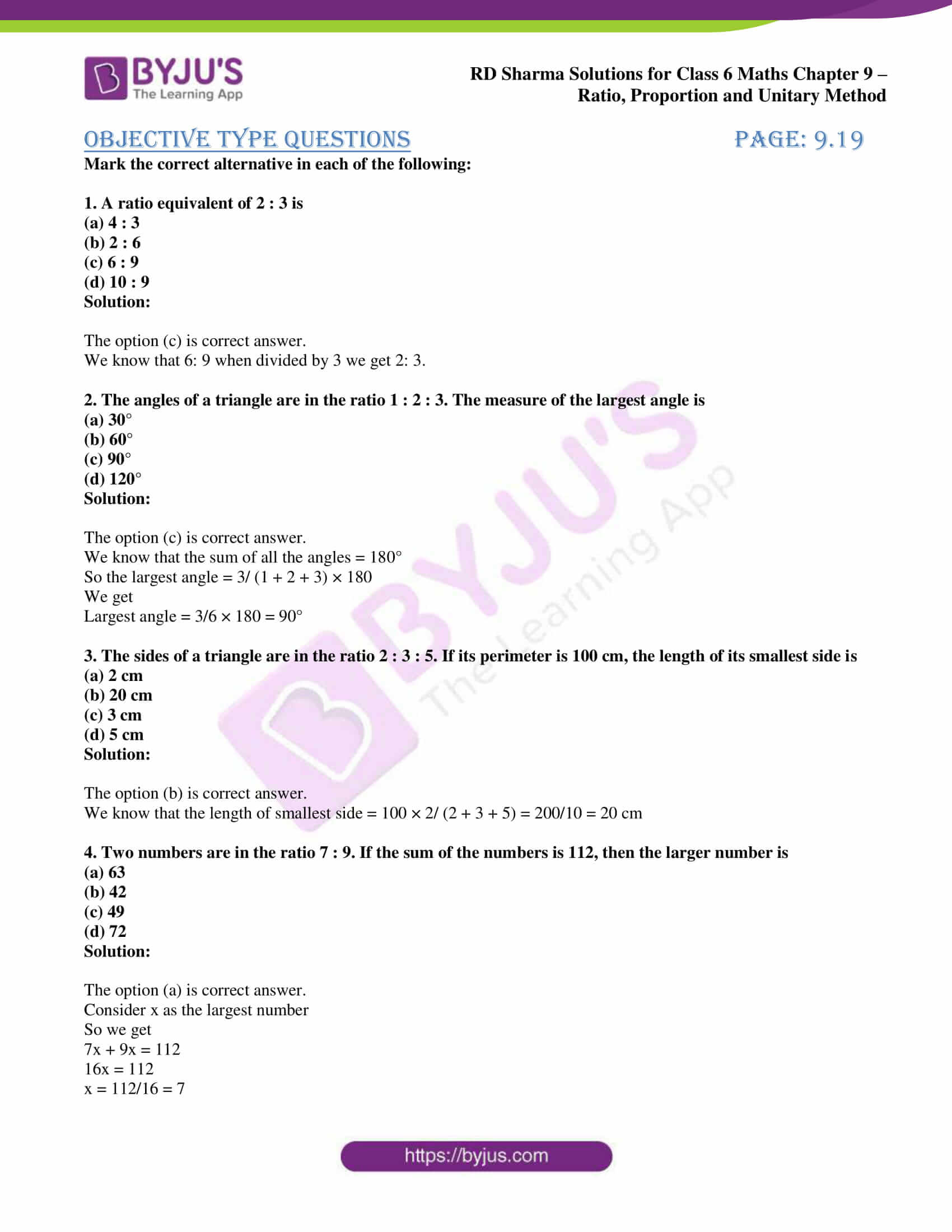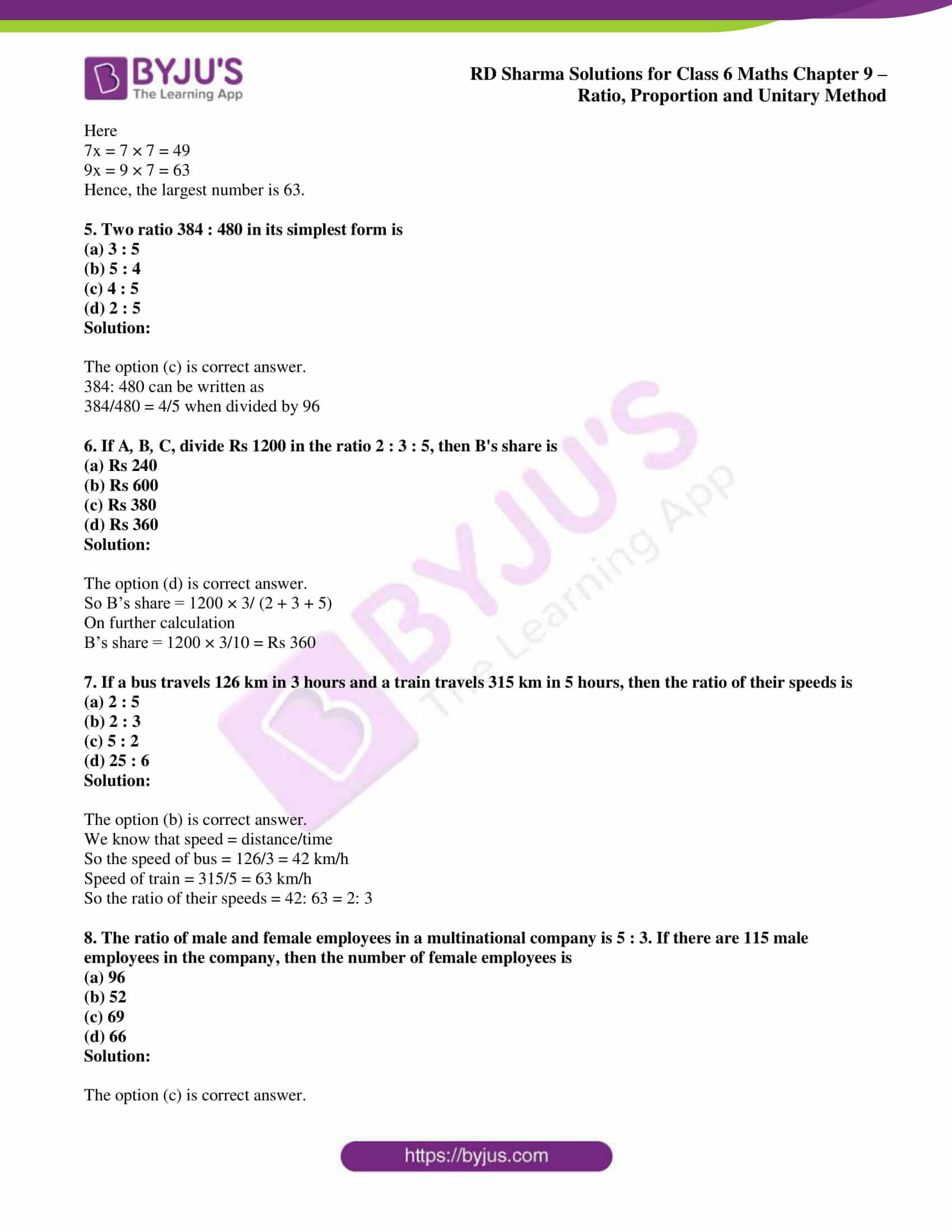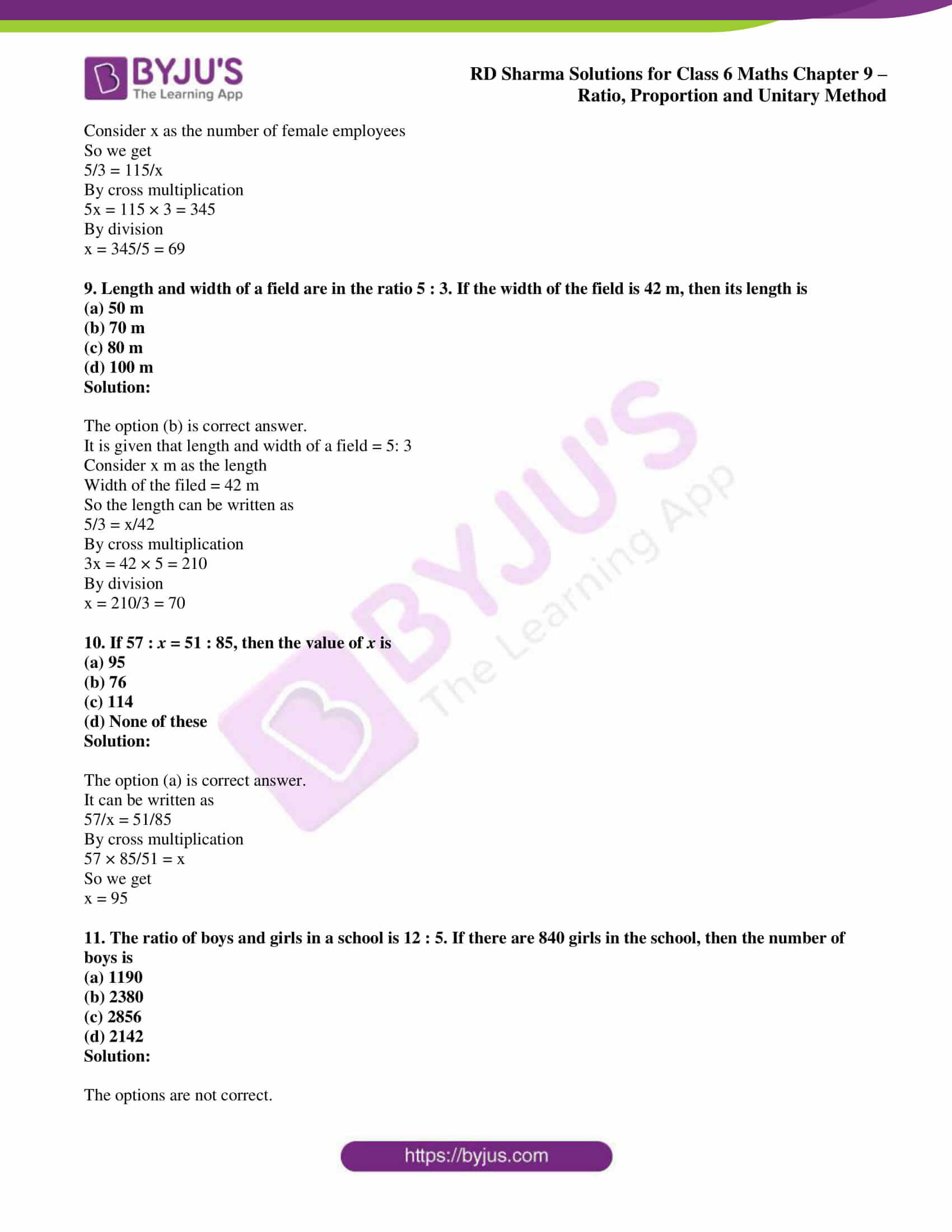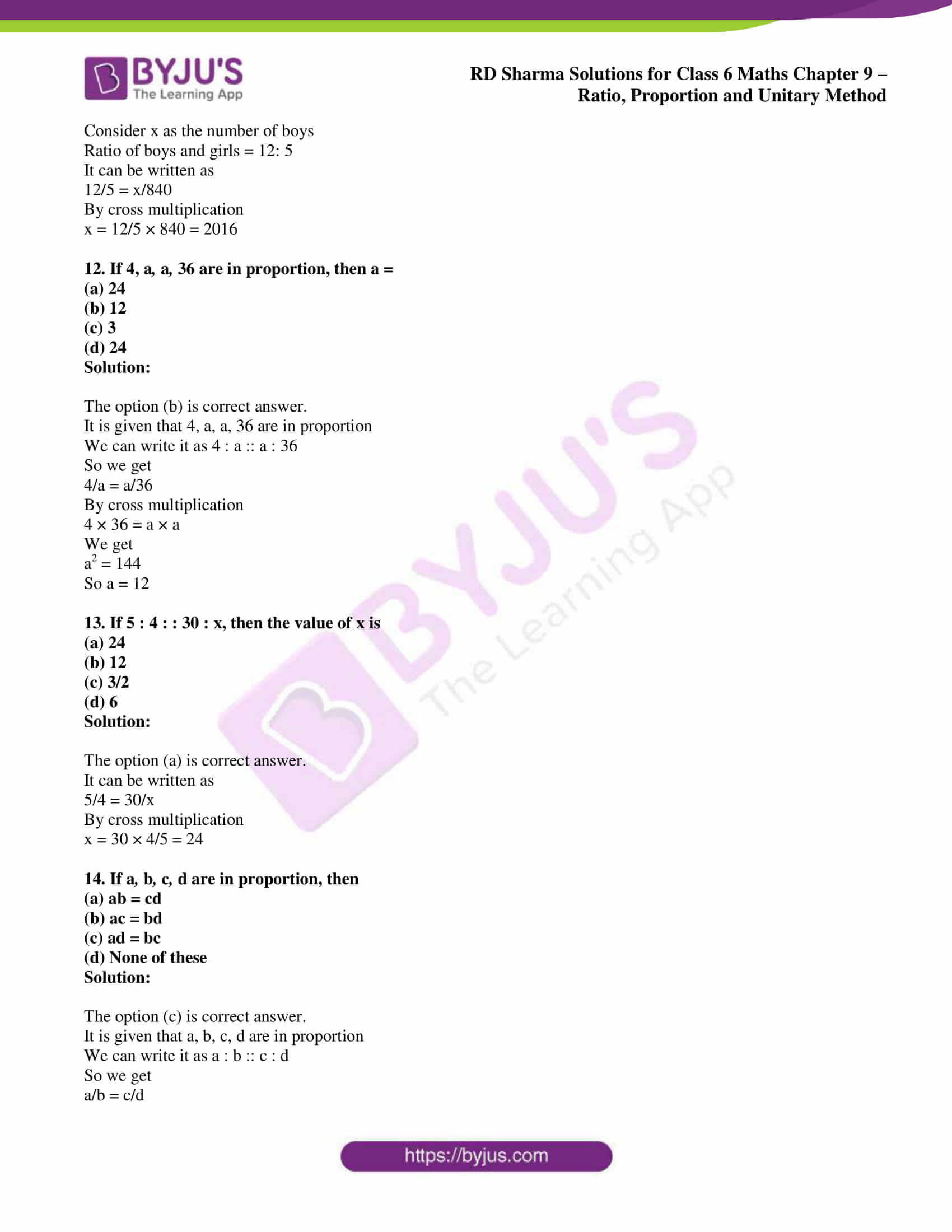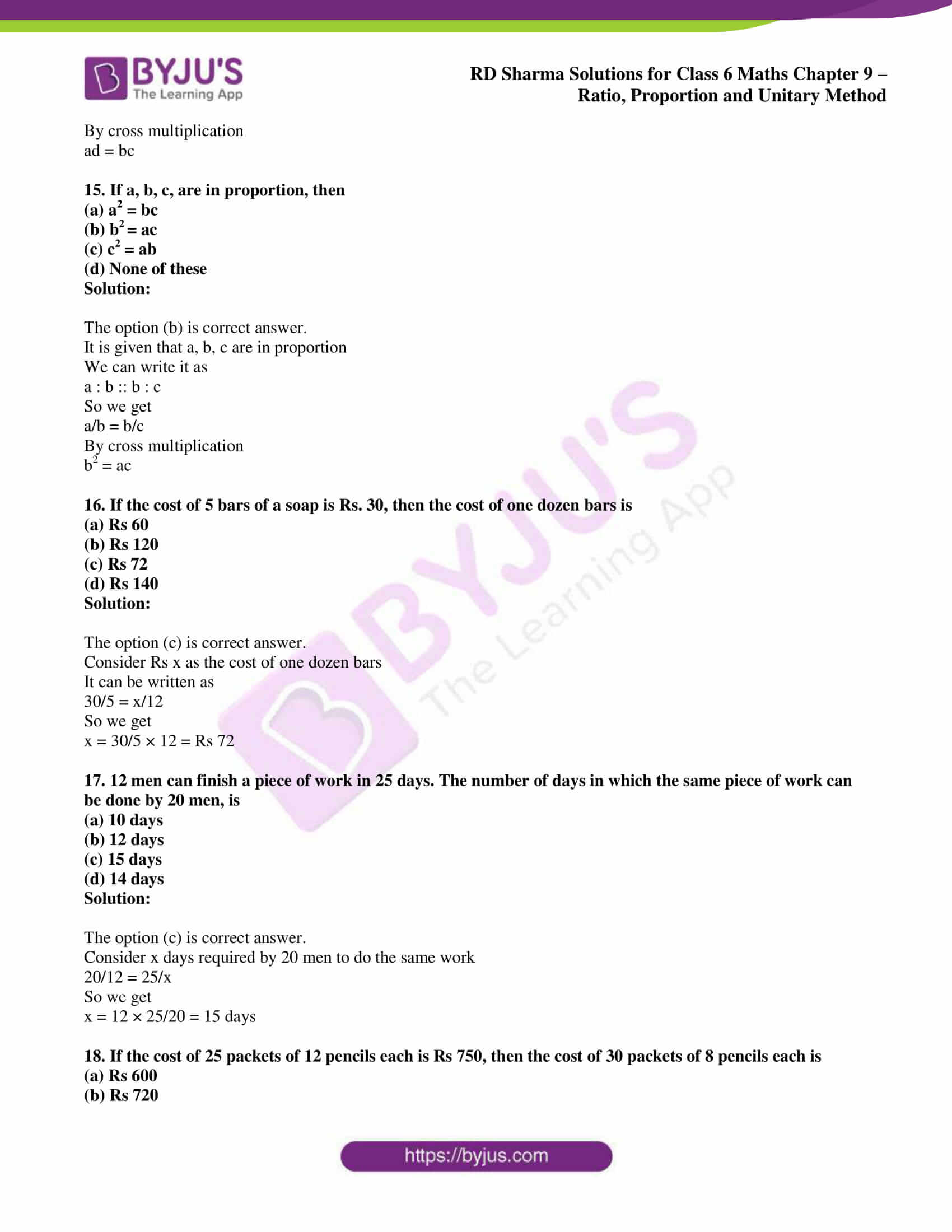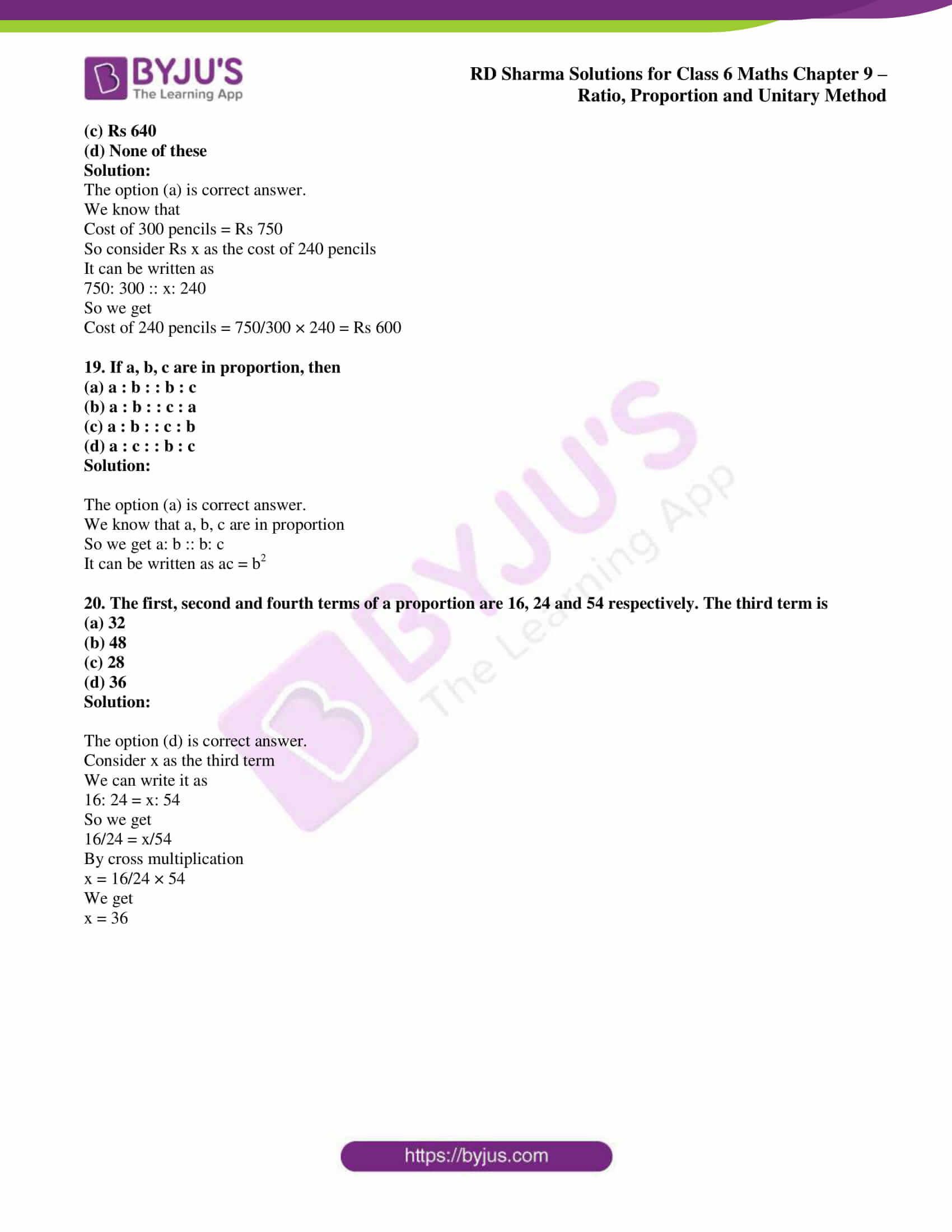### Access answers to Maths RD Sharma Solutions for Class 6 Chapter 9: Ratio, Proportion and Unitary Method Objective Type Questions

Mark the correct alternative in each of the following:

1. A ratio equivalent of 2 : 3 is
(a) 4 : 3
(b) 2 : 6
(c) 6 : 9
(d) 10 : 9

Solution:

The option (c) is correct answer.

We know that 6: 9 when divided by 3 we get 2: 3.

2. The angles of a triangle are in the ratio 1 : 2 : 3. The measure of the largest angle is
(a) 30°
(b) 60°
(c) 90°
(d) 120°

Solution:

The option (c) is correct answer.

We know that the sum of all the angles = 180°

So the largest angle = 3/ (1 + 2 + 3) × 180

We get

Largest angle = 3/6 × 180 = 90°

3. The sides of a triangle are in the ratio 2 : 3 : 5. If its perimeter is 100 cm, the length of its smallest side is
(a) 2 cm
(b) 20 cm
(c) 3 cm
(d) 5 cm

Solution:

The option (b) is correct answer.

We know that the length of smallest side = 100 × 2/ (2 + 3 + 5) = 200/10 = 20 cm

4. Two numbers are in the ratio 7 : 9. If the sum of the numbers is 112, then the larger number is
(a) 63
(b) 42
(c) 49
(d) 72

Solution:

The option (a) is correct answer.

Consider x as the largest number

So we get

7x + 9x = 112

16x = 112

x = 112/16 = 7

Here

7x = 7 × 7 = 49

9x = 9 × 7 = 63

Hence, the largest number is 63.

5. Two ratio 384 : 480 in its simplest form is
(a) 3 : 5
(b) 5 : 4
(c) 4 : 5
(d) 2 : 5

Solution:

The option (c) is correct answer.

384: 480 can be written as

384/480 = 4/5 when divided by 96

6. If ABC, divide Rs 1200 in the ratio 2 : 3 : 5, then B’s share is
(a) Rs 240
(b) Rs 600
(c) Rs 380
(d) Rs 360

Solution:

The option (d) is correct answer.

So B’s share = 1200 × 3/ (2 + 3 + 5)

On further calculation

B’s share = 1200 × 3/10 = Rs 360

7. If a bus travels 126 km in 3 hours and a train travels 315 km in 5 hours, then the ratio of their speeds is
(a) 2 : 5
(b) 2 : 3
(c) 5 : 2
(d) 25 : 6

Solution:

The option (b) is correct answer.

We know that speed = distance/time

So the speed of bus = 126/3 = 42 km/h

Speed of train = 315/5 = 63 km/h

So the ratio of their speeds = 42: 63 = 2: 3

8. The ratio of male and female employees in a multinational company is 5 : 3. If there are 115 male employees in the company, then the number of female employees is
(a) 96
(b) 52
(c) 69
(d) 66

Solution:

The option (c) is correct answer.

Consider x as the number of female employees

So we get

5/3 = 115/x

By cross multiplication

5x = 115 × 3 = 345

By division

x = 345/5 = 69

9. Length and width of a field are in the ratio 5 : 3. If the width of the field is 42 m, then its length is
(a) 50 m
(b) 70 m
(c) 80 m
(d) 100 m

Solution:

The option (b) is correct answer.

It is given that length and width of a field = 5: 3

Consider x m as the length

Width of the filed = 42 m

So the length can be written as

5/3 = x/42

By cross multiplication

3x = 42 × 5 = 210

By division

x = 210/3 = 70

10. If 57 : x = 51 : 85, then the value of x is
(a) 95
(b) 76
(c) 114
(d) None of these

Solution:

The option (a) is correct answer.

It can be written as

57/x = 51/85

By cross multiplication

57 × 85/51 = x

So we get

x = 95

11. The ratio of boys and girls in a school is 12 : 5. If there are 840 girls in the school, then the number of boys is
(a) 1190
(b) 2380
(c) 2856
(d) 2142

Solution:

The options are not correct.

Consider x as the number of boys

Ratio of boys and girls = 12: 5

It can be written as

12/5 = x/840

By cross multiplication

x = 12/5 × 840 = 2016

12. If 4, aa, 36 are in proportion, then a =
(a) 24
(b) 12
(c) 3
(d) 24

Solution:

The option (b) is correct answer.

It is given that 4, a, a, 36 are in proportion

We can write it as 4 : a :: a : 36

So we get

4/a = a/36

By cross multiplication

4 × 36 = a × a

We get

a2 = 144

So a = 12

13. If 5 : 4 : : 30 : x, then the value of x is
(a) 24
(b) 12
(c) 3/2
(d) 6

Solution:

The option (a) is correct answer.

It can be written as

5/4 = 30/x

By cross multiplication

x = 30 × 4/5 = 24

14. If abcd are in proportion, then
(a) ab = cd
(b) ac = bd
(d) None of these

Solution:

The option (c) is correct answer.

It is given that a, b, c, d are in proportion

We can write it as a : b :: c : d

So we get

a/b = c/d

By cross multiplication

15. If a, b, c, are in proportion, then
(a) a2 = bc
(b) b= ac
(c) c2 = ab
(d) None of these

Solution:

The option (b) is correct answer.

It is given that a, b, c are in proportion

We can write it as

a : b :: b : c

So we get

a/b = b/c

By cross multiplication

b2 = ac

16. If the cost of 5 bars of a soap is Rs. 30, then the cost of one dozen bars is
(a) Rs 60
(b) Rs 120
(c) Rs 72
(d) Rs 140

Solution:

The option (c) is correct answer.

Consider Rs x as the cost of one dozen bars

It can be written as

30/5 = x/12

So we get

x = 30/5 × 12 = Rs 72

17. 12 men can finish a piece of work in 25 days. The number of days in which the same piece of work can be done by 20 men, is
(a) 10 days
(b) 12 days
(c) 15 days
(d) 14 days

Solution:

The option (c) is correct answer.

Consider x days required by 20 men to do the same work

20/12 = 25/x

So we get

x = 12 × 25/20 = 15 days

18. If the cost of 25 packets of 12 pencils each is Rs 750, then the cost of 30 packets of 8 pencils each is

(a) Rs 600

(b) Rs 720

(c) Rs 640

(d) None of these

Solution:

The option (a) is correct answer.

We know that

Cost of 300 pencils = Rs 750

So consider Rs x as the cost of 240 pencils

It can be written as

750: 300 :: x: 240

So we get

Cost of 240 pencils = 750/300 × 240 = Rs 600

19. If a, b, c are in proportion, then
(a) a : b : : b : c
(b) a : b : : c : a
(c) a : b : : c : b
(d) a : c : : b : c

Solution:

The option (a) is correct answer.

We know that a, b, c are in proportion

So we get a: b :: b: c

It can be written as ac = b2

20. The first, second and fourth terms of a proportion are 16, 24 and 54 respectively. The third term is
(a) 32
(b) 48
(c) 28
(d) 36

Solution:

The option (d) is correct answer.

Consider x as the third term

We can write it as

16: 24 = x: 54

So we get

16/24 = x/54

By cross multiplication

x = 16/24 × 54

We get

x = 36